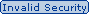#### Which of the following equations results in the Single Loss Expectancy for an asset?Send Via Email (Need Reachable Email ID)## 99.9% OFF All In One Supper Package

Which of the following equations results in the Single Loss Expectancy for an asset?
A. Asset Value x % Of Loss From Realized Exposure
B. Asset Value x % Of Loss From Realized Threat
C. Annualized Rate of Occurrence / Annualized Loss Expectancy
D. Asset Value x % Of Loss From Realized Vulnerability
E. Annualized Rate of Occurrence x Annualized Loss Expectancy